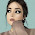Given two words (start and end), and a dictionary, find the length of shortest transformation sequence from start to end, such that only one letter can be changed at a time and each intermediate word must exist in the dictionary. For example, given:

start = "hit"
end = "cog"
dict = ["hot","dot","dog","lot","log"]
One shortest transformation is "hit" -> "hot" -> "dot" -> "dog" -> "cog", the program should return its length 5.

Note:
Return 0 if there is no such transformation sequence.
All words have the same length.
All words contain only lowercase alphabetic characters.

### Using Breadth First Search

Here we have to use One Queue for storing words and One Queue for storing distances.
1. Add start-word to word-queue, add 1 to distance queue, Add end word to dictionary
2. Pop word from word-queue and use it as start word, pop distance from distance-queue and use it as current distance
3. Replace each character of start word and verify in dictionary. If the word is there in dictionary then add that word to word-queue and increment length and add length to distance-queue. Remove that word from dictionary
4. Repeat above 2 steps until dictionary become empty.
5. Return current distance as resultant distance

```function ladderLength2(start, end, dict) {
if (dict.length == 0)
return 0;

dict.push(end);

var wordQueue = [];
var distanceQueue = [];

wordQueue.push(start);
distanceQueue.push(1);

//track the shortest path
var result = 9007199254740992;
while (wordQueue.length != 0) {
var currWord = wordQueue.pop();
var currDistance = distanceQueue.pop();

if (currWord == end) {
result = Math.min(result, currDistance);
}

for (var i = 0; i < currWord.length; i++) {
var currCharArr = currWord.split('');
for (var c = 'a'; c <= 'z'; c = nextChar(c)) {
currCharArr[i] = c;
var newWord = currCharArr.join('');
if (dict.indexOf(newWord) != -1) {
wordQueue.push(newWord);
distanceQueue.push(currDistance + 1);
var index = dict.indexOf(newWord);
if (index > -1) {
dict.splice(index, 1);
}
}
}
}
}

if (result < 9007199254740992)
return result;
else
return 0;
}

function nextChar(c) {
return String.fromCharCode(c.charCodeAt(0) + 1);
}
```

1.Should be 5 instead of 7 for mentioned above testcaseЖ

2.💰Get free 250\$ on Ysense trusted network without investment

📢 Join Crypto quantum leap world's famous online Crypto course

🦷 Read Secret teeth care tips
Teeth whitening,
Teeth decay,
Teethsche,

## BlogrollSrinivas Dasari find me on facebook follow me on twitter